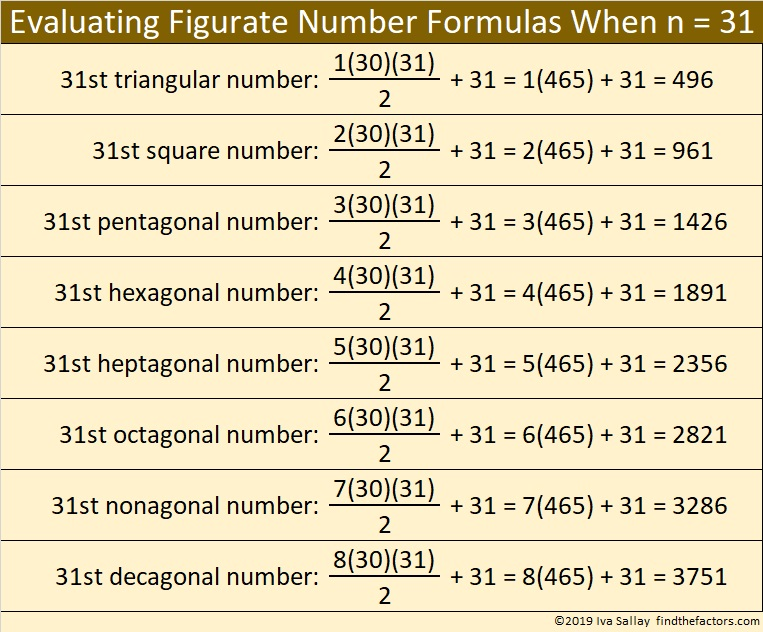# 1426 is a Pentagonal Number

I remembered that 1426 is a pentagonal number, but I didn’t have the formula for pentagonal numbers memorized. While I was waiting to do something else, I tried to come up with the formula myself.

The formula I derived isn’t what you usually see, but I rather like it! This formula can be extended to any figurate number as the chart below shows.I love that the distance between consecutive figurate numbers on the chart is 465 which is the 30th triangular number!

Now if you asked me how many little dots does the 31st 1000-gonal number have, I also would be able to tell you that it has 998(465) + 31 = 464101 dots!

But today’s post is asking for the 31st pentagonal number. Here is what that looks like:Can you see that it is three times the 30th triangular number plus 31?

Here are some more facts about the number 1426:

• 1426 is a composite number.
• Prime factorization: 1426 = 2 × 23 × 31.
• 1426 has no exponents greater than 1 in its prime factorization, so √1426 cannot be simplified.
• The exponents in the prime factorization are 1, 1, and 1. Adding one to each exponent and multiplying we get (1 + 1)(1 + 1)(1 + 1) = 2 × 2 × 2 = 8. Therefore 1426 has exactly 8 factors.
• The factors of 1426 are outlined with their factor pair partners in the graphic below.Since 1426 = 2(31)(23) and 2(713)(1), it is a leg in two Pythagorean triples:
432-1426-1490 calculated from 31² – 23², 2(31)(23), 31² + 23²
1426-508368-508370 calculated from 2(713)(1), 713² – 1², 713² + 1²

This site uses Akismet to reduce spam. Learn how your comment data is processed.